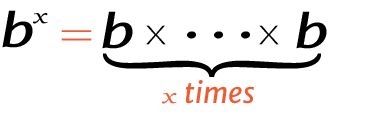# 3 raise to the power of 7

Place the base and exponent in the power calculator to get the right answer for value of 7^3, 73 (7 power 3), or 3 raised to the power of 7.

## How to calculate 7 raise to the power of 3?

This is how you can solve (7) 3 manually.

Solution:

Step 1: Simplify the data.

Base
ExponentStep 2: Multiply the 7 to itself 3 times.

Using this exponents calculator could be much easier to figure out an exponential expression.